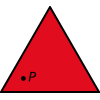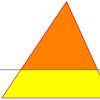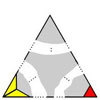Search by Topic

Resources tagged with Triangles similar to Biggest Bendy:

Filter by: Content type:
Age range:
Challenge level:

There are 26 results

Broad Topics > Angles, Polygons, and Geometrical Proof > TrianglesHexy-metry

Age 14 to 16 Challenge Level:

A hexagon, with sides alternately a and b units in length, is inscribed in a circle. How big is the radius of the circle?Three Way Split

Age 14 to 16 Challenge Level:

Take any point P inside an equilateral triangle. Draw PA, PB and PC from P perpendicular to the sides of the triangle where A, B and C are points on the sides. Prove that PA + PB + PC is a constant.Xtra

Age 14 to 18 Challenge Level:

Find the sides of an equilateral triangle ABC where a trapezium BCPQ is drawn with BP=CQ=2 , PQ=1 and AP+AQ=sqrt7 . Note: there are 2 possible interpretations.Incircles Explained

Age 16 to 18Age 14 to 16 Challenge Level:

The sides of a triangle are 25, 39 and 40 units of length. Find the diameter of the circumscribed circle.Are You Kidding

Age 14 to 16 Challenge Level:

If the altitude of an isosceles triangle is 8 units and the perimeter of the triangle is 32 units.... What is the area of the triangle?Calculating with Cosines

Age 14 to 18 Challenge Level:

If I tell you two sides of a right-angled triangle, you can easily work out the third. But what if the angle between the two sides is not a right angle?Half a Triangle

Age 14 to 16 Challenge Level:

Construct a line parallel to one side of a triangle so that the triangle is divided into two equal areas.Triangle in a Triangle

Age 14 to 16 Challenge Level:

Can you work out the fraction of the original triangle that is covered by the inner triangle?Fitting In

Age 14 to 16 Challenge Level:

The largest square which fits into a circle is ABCD and EFGH is a square with G and H on the line CD and E and F on the circumference of the circle. Show that AB = 5EF. Similarly the largest. . . .Arrh!

Age 14 to 16 Challenge Level:

Triangle ABC is equilateral. D, the midpoint of BC, is the centre of the semi-circle whose radius is R which touches AB and AC, as well as a smaller circle with radius r which also touches AB and AC. . . .Pareq Calc

Age 14 to 16 Challenge Level:

Triangle ABC is an equilateral triangle with three parallel lines going through the vertices. Calculate the length of the sides of the triangle if the perpendicular distances between the parallel. . . .Lens Angle

Age 14 to 16 Challenge Level:

Find the missing angle between the two secants to the circle when the two angles at the centre subtended by the arcs created by the intersections of the secants and the circle are 50 and 120 degrees.Tricircle

Age 14 to 16 Challenge Level:

The centre of the larger circle is at the midpoint of one side of an equilateral triangle and the circle touches the other two sides of the triangle. A smaller circle touches the larger circle and. . . .Area I'n It

Age 16 to 18 Challenge Level:

Triangle ABC has altitudes h1, h2 and h3. The radius of the inscribed circle is r, while the radii of the escribed circles are r1, r2 and r3 respectively. Prove: 1/r = 1/h1 + 1/h2 + 1/h3 = 1/r1 +. . . .Age 14 to 16 Challenge Level:

Find the area of the shaded region created by the two overlapping triangles in terms of a and b?Equal Equilateral Triangles

Age 14 to 16 Challenge Level:

Using the interactivity, can you make a regular hexagon from yellow triangles the same size as a regular hexagon made from green triangles ?Impossible Triangles?

Age 16 to 18 Challenge Level:

Which of these triangular jigsaws are impossible to finish?Farhan's Poor Square

Age 14 to 16 Challenge Level:

From the measurements and the clue given find the area of the square that is not covered by the triangle and the circle.Pareq Exists

Age 14 to 16 Challenge Level:

Prove that, given any three parallel lines, an equilateral triangle always exists with one vertex on each of the three lines.The Square Hole

Age 14 to 16 Challenge Level:

If the yellow equilateral triangle is taken as the unit for area, what size is the hole ?Interacting with the Geometry of the Circle

Age 5 to 16

Jennifer Piggott and Charlie Gilderdale describe a free interactive circular geoboard environment that can lead learners to pose mathematical questions.Triangle Midpoints

Age 14 to 16 Challenge Level:

You are only given the three midpoints of the sides of a triangle. How can you construct the original triangle?Two Triangles in a Square

Age 14 to 16 Challenge Level:

Given that ABCD is a square, M is the mid point of AD and CP is perpendicular to MB with P on MB, prove DP = DC.Wedge on Wedge

Age 14 to 16 Challenge Level:

Two right-angled triangles are connected together as part of a structure. An object is dropped from the top of the green triangle where does it pass the base of the blue triangle?LOGO Challenge 5 - Patch

Age 11 to 16 Challenge Level:

Using LOGO, can you construct elegant procedures that will draw this family of 'floor coverings'?